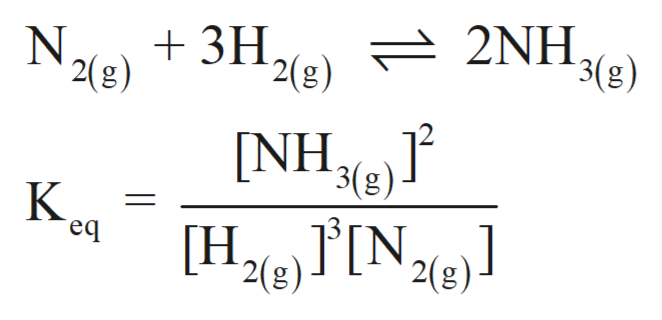Consider the following chemical equilibrium:N2(g) + 3H2(g) ⇌ 2NH3(g)Now write an equation below that shows how to calculate Kc from Kp for this reaction at an absolute temperature T. You can assume T is comfortably above room temperature. If you include any common physical constants in your equation be sure you use their standard symbols, found in the ALEKS Calculator.

Question

Consider the following chemical equilibrium:

N2(g) + 3H2(g) ⇌ 2NH3(g)

Now write an equation below that shows how to calculate Kc from Kp for this reaction at an absolute temperature T. You can assume T is comfortably above room temperature. If you include any common physical constants in your equation be sure you use their standard symbols, found in the ALEKS Calculator.

Step 1

A reversible chemical reaction can move in either forward or backward direction. The stage of a reversible reaction at which the rate of forward and backward reaction become equal to each other is called an equilibrium stage.

Step 2

The equilibrium constant for a reaction is the ratio of equilibrium concentration of product and reactant. If the reaction is given in gaseous state, then the equilibrium pressure must be considered.

Step 3

The Keq expression for the below...help_outlineImage Transcriptionclose2(g) 2NH. 3(g) 3H N 2(g) [NH 3(g) K H2 IN2e] eq 2(g fullscreen

Want to see the full answer?

See Solution

Want to see this answer and more?

Our solutions are written by experts, many with advanced degrees, and available 24/7

See Solution
Tagged in

Chemistry